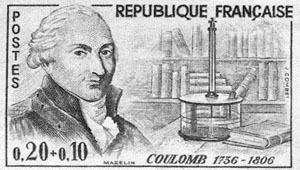# Charles Coulomb: Discoveries & Overview

Instructor: Jeff Fennell

Jeff has a master's in engineering and has taught Earth science both domestically and internationally.

Charles Coulomb (June 14, 1736 - August 23, 1806) was a French physicist and military engineer. This lesson will cover his scientific achievements. After the lesson, take the quiz to test your knowledge.

## Discovery

Coulomb is most famous for his work with electricity and magnetism. His studies and papers were the first thorough accounts of how electricity worked and its relationship to magnetism.

In 1777, Coulomb understood that a needle on a pin creates friction and reduces accuracy. Coulomb replaced the fulcrum with a fine silk thread, and rather than the up-and-down motion of the pan balance, he used a twist or torsion around this thread. He was able to show that the amount of torsion is proportional to the amount of force.## Electromagnetism

Between 1785 and 1789, Coulomb studied the force of charges through precise experiments at the Royal Academy and published numerous papers on his findings. In 1785, Coulomb presented three papers on electricity and magnetism. These papers led Coulomb to the law that would eventually bear his name, Coulomb's law. Coulomb's law was the first dealing with a quantitative analysis of electricity.

Premier Mémoire sur l'Électricité et le Magnétisme

In his first paper, Coulomb describes the technique to construct his torsion balance, and the properties of the metal wires of having a reaction torsion force proportional to the torsion angle.' In this paper, Coulomb derived the law two bodies electrified of the same kind of electricity exert a repulsive force on each other.

Second Mémoire sur l'Électricité et le Magnétisme

In this publication, Coulomb describes the attractive force between two oppositely-charged objects. The force is proportional to the product of the quantities of charge on the objects and inversely proportional to the square of the distance between the objects.

Troisième Mémoire sur l'Électricité et le Magnétisme

In his second paper, Coulomb describes the loss of electricity that an isolated body loses over time.

### Coulomb's Law

Coulomb's law states that in a vacuum, the force between two stationary point charges is inversely proportional to the square of the distance. The power is proportional to the product of the force in the direction in which connected. Charges of the same sign repel, while opposite charge attract.

Mathematically written, Coulomb's law states:

F = k * (q1 * q2) / r ^ 2.

Where:

• F is the electrostatic force between the charges
• r is the distance between q1 and q2
• k is Coulomb's constant = 8.897 x 10^9 N m^2 C^-2
• q1 is charge 1
• q2 is charge 2

To unlock this lesson you must be a Study.com Member.

### Register to view this lesson

Are you a student or a teacher?